Published: 15 August 2017

# Effect of the blade outlet angle on unsteady characteristics of a single channel pump

Minggao Tan1
Zhipeng Zhu2
Houlin Liu3
Xianfang Wu4
Jinsheng Feng5
1, 2, 3, 5Research Center of Fluid Machinery Engineering and Technology, Jiangsu University, Zhenjiang, China
4School of Energy and Power Engineering, Jiangsu University, Zhenjiang, China
Corresponding Author:
Zhipeng Zhu
Views 110

#### Abstract

To study the effect of ${\beta }_{2}$ on energy performance and unsteady characteristics of the single-channel pump, the experimental tests about the energy characteristics, head pulsation, pressure fluctuation and radial force have been conducted by the synchronous test. 3 different impellers with the blade outlet angle ${\beta }_{2}$ of 8°, 16° and 25° respectively are studied. The results show that with the increase of ${\beta }_{2}$ from 8° to 25°, the head increases gradually and the maximum increase amplitude reaches 22.6 %. As ${\beta }_{2}$ changes from 8° to 25°, there is a maximum efficiency. The mixing loss at the impeller outlet can be decreased by reducing ${\beta }_{2}$. With the increasing of ${\beta }_{2}$, the minimum head in time domain gradually lag and the maximum head gradually advances. The pressure fluctuation in each measuring point shows the trend of increasing first and then decreasing with the increasing of the flow rate. With ${\beta }_{2}$ increasing, the radial force also increases and the maximum increase amplitude of minimum radial force is larger than 8 %. The research can provide some reference for the optimization of single channel pumps.

## 1. Introduction

As the favorable no-clogging performance of the single channel pump, it has been the key facilities in sewage treatment. However, due to the highly asymmetry structure of the single channel impeller, the unsteady characteristics is obvious during its operation, such as huge head fluctuation and radial force pulsation. Therefore, it is important to control instability of the single channel pump.

Berna  simulated the inner flow in a single blade pump, and the results show that the head pulsation increases with the increasing of flow rate. At different flow rate, the maximum head and the minimum head all appear at the same impeller rotation position. Daly  simulated the inner flow in the single blade pump. The results show that the blade position has little effect on pressure distribution in impeller, while the pressure distribution in volute changes obviously with the impeller rotating. The high pressure domain appears at the downstream of blade outlet edge and the low pressure domain appears at upstream of blade outlet edge, which agrees well with the head pulsation. Siekmann  studied the effect of wrap angle (from 289° to 420°) on the radial force in a single blade pump and found that with the increase of wrap angle the radial force gradually increases at 0.5${Q}_{d}$ and gradually decreases at the design and large flow rate. Nishi [4-6] studied the radial force of the single channel impeller by experiments carefully and the results show that the radial force mainly comes from flow induced force on impeller.

The research above mainly focused on the head pulsation, pressure fluctuation and radial force of the pump and the geometric parameters have obvious influence on the performance of the single channel pump.

What is well known that the influence of the ${\beta }_{2}$ on the energy characteristic and inner flow is pretty complex. Wu  studied the effect of the ${\beta }_{2}$ on the performance curve of centrifugal pump and indicated that the ${\beta }_{2}$ has more effect on high specific speed centrifugal pump. Tan  researched the effect of the blade outlet angel on the energy performance and indicated that the ${\beta }_{2}$ has an importance effect on the jet-wake structure in the centrifugal pump. So, the ${\beta }_{2}$ has more effect on the middle and high speed centrifugal pumps. Therefore, the ${\beta }_{2}$ as a significant parameter of single channel pump is set as the study variable.

Hence, a single channel pump, whose specific speed is 140, is selected as the research model and a synchronous experiment rig was built to reveal the influence of the ${\beta }_{2}$ on the energy performance and the unsteady characteristics of the single channel pumps. The research findings can be set as the foundation of establishing the design method for unsteady reliability of the single channel impeller applied to engineering practice by further research.

## 2. Test model and rig and scheme

### 2.1. Test model

The main geometrical and hydraulic data of the model pump are displayed in Table1.

### 2.2. The test rig

It is shown as the Fig. 1 that an opened-loop test rig was conducted to test the energy performance and unsteady characteristics of the single channel pump. The tested pump was driven by a variable speed electric AC submersible motor controlled by a frequency converter. The test apparatus includes the magnetic flow meter, Hall induction coil, three-phase power acquisition instrument, static pressure transfer, pump parameters acquisition instrument and pump parameter analysis software.

The pressure measuring points were distributed at impeller outlet to obtain the unsteady characteristics of the pump . As shown the Fig. 2, the 16 pressure measuring points were set at the impeller outlet in an absolute coordinate and the angle between two consecutive measuring points is 22.5°, and P1 is at $\alpha =$ 0°. The measuring points on the model pump are presented in Fig. 3. Pressure fluctuation was measured by the waterproof pressure transducers (HY6305 series) at P2, P6, P10 and P14. And the other 12 monitoring points was connected by flexible pipes and used to measure pressure.

Table 1Main geometric parameters and hydraulic data

 Geometric parameters Symbol Value The impeller inlet diameter (mm) ${D}_{j}$ 125 The impeller outlet diameter (mm) ${D}_{2}$ 300 The impeller outlet width (mm) ${b}_{2}$ 100 The blade inlet thickness (mm) ${\delta }_{1}$ 10.5 The blade outlet thickness (mm) ${\delta }_{2}$ 5.15 Blade wrap angle ${\epsilon }_{sb}$ 340° The blade inlet width (mm) ${b}_{1}$ 115 Design parameters Flow rate (m3/h) ${Q}_{d}$ 220 Head (m) ${H}_{d}$ 20 Rotational speed (r/min) $n$ 1474

Fig. 1Test rig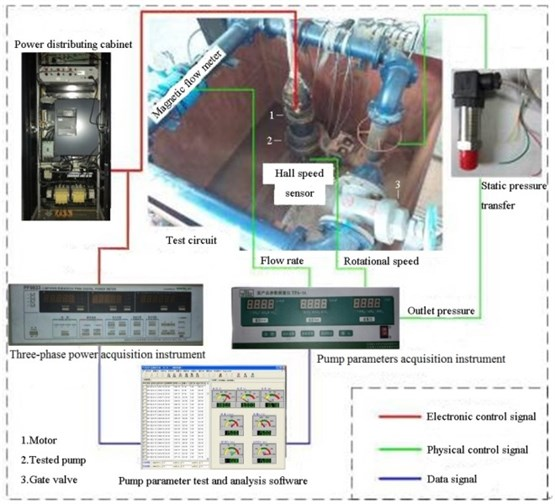Fig. 2The coordinate and measuring points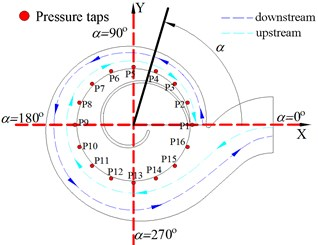Fig. 3The measuring points on the model pump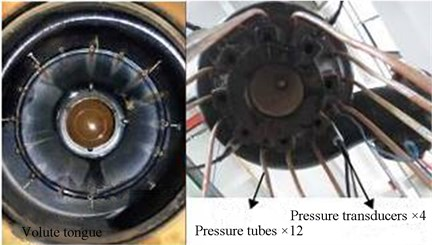The impeller position was monitored by Hall sensor [10, 11]. As shown in Fig. 4, the sensor was installed at the volute tongue and the inductive magnet was fixed on the blade outlet edge. When the blade outlet passes the tongue, the Hall sensor gives a square pulse signal. To show impeller position conveniently, $\theta$ is applied to indicate the impeller rotation angle. The impeller rotation angle $\theta$ is 0° when blade outlet edge just sweeps across the tongue.

Fig. 4The installation of Hall sensor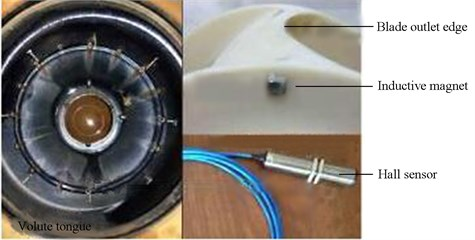### 2.3. Test schemes

To study the effect of the ${\beta }_{2}$ on the energy performance and unsteady characteristics, 3 schemes were designed, namely the ${\beta }_{2}$ is 8°, 16° and 25° respectively. Three impeller models are shown in Fig. 5.

Fig. 5Impellers with different blade outlet angle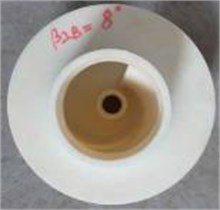a)${\beta }_{2}=$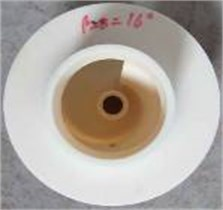b)${\beta }_{2}=$ 16°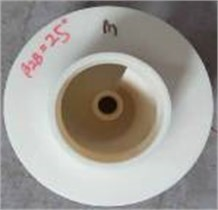c)${\beta }_{2}=$ 25°

## 3. Results and discussion

### 3.1. The effect on the energy characteristics

The pump head and efficiency under different blade outlet angles are shown in Fig. 6.

From Fig. 6(a), it can be seen that pump head all decreases gradually with the increase of flow rate under different ${\beta }_{2}$. With the increase of ${\beta }_{2}$, the pump head gets bigger. What is obvious is that the increase amplitude is different with the variation of ${\beta }_{2}$. When the ${\beta }_{2}$ changes from 8° to 16°, the increase amplitude of head at design flow rate is 15.7 %, while it is 22.3 % as the ${\beta }_{2}$ varies from 16° to 25°. Therefore, the increase amplitude of head gradually decreases as ${\beta }_{2}$ increases.

It can be found from the Fig. 6(b) that pump efficiency reaches the maximum while the flow rate coefficients respectively is 0.0306, 0.0345, 0.0321, corresponding to 1.09${Q}_{d}$, 1.23${Q}_{d}$ and 1.15${Q}_{d}$, under ${\beta }_{2}$ of 8°, 16° and 25°. At the design flow rate and large flow rate, the effect of ${\beta }_{2}$ on efficiency is more obvious. As the ${\beta }_{2}$ varies from 8° to 16°, under all flow rates the efficiency increases. In this case, the maximum efficiency increases 6.48 percentages. When the ${\beta }_{2}$ changes from 16° to 25°, under all flow rates the efficiency decreases. Under the circumstances, the maximum efficiency decreases 2.18 percentages. At small flow rate, even the efficiency of pump with ${\beta }_{2}$ of 25° is smaller than that of pump with ${\beta }_{2}$ of 16°. So, the effect of ${\beta }_{2}$ on pump efficiency is more complicate than that on pump head.

The explanation for this phenomenon may be the diversity effect of ${\beta }_{2}$. With the increase of ${\beta }_{2}$, although the pump head would increase, the hydraulic loss of flow separation and shock also would increase.

Fig. 6The performance curves under different β2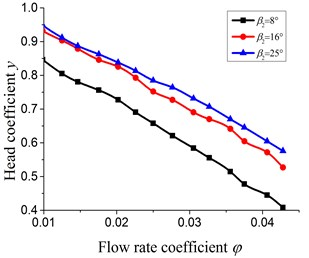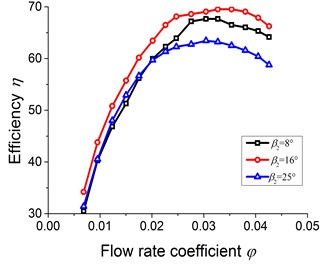b) The efficiency curves

### 3.2. The effect on the mixing loss

Because the ${b}_{2}/{D}_{2}$ of the single channel impeller is far greater than the multi-blade centrifugal pump, the uniformity of the velocity and pressure at the impeller outlet isn’t good obviously, which means the maxing loss at the impeller outlet is huge. The static pressure distribution at impeller outlet can be used to represent the mixing loss between impeller outlet and volute inlet. The more uniform the static pressure distribution is, the less the mixing loss is [12, 13]. So, the static pressure distribution is tested by the 16 measuring points at the impeller outlet. To analyze conveniently, non-dimensional treatment for pressure signal was applied, and the formula is ${P}_{A}=2p/\rho {u}_{2}^{2}$.

Fig. 7 shows the static pressure distribution at impeller outlet under 0.6${Q}_{d}$, 1.0${Q}_{d}$ and 1.4${Q}_{d}$. It is seen that at 0.6${Q}_{d}$, the maximum pressure all arises at 45° under different ${\beta }_{2}$ and under ${\beta }_{2}$ of 8°, 16° and 25°, the maximum pressure is 0.66, 0.72 and 0.75 respectively. At 1.0${Q}_{d}$, the maximum pressure appears between 22.5° and 45° and under ${\beta }_{2}$ of 8°, 16° and 25°, the maximum pressure is 0.54, 0.60 and 0.65 respectively. At 1.4${Q}_{d}$, the maximum pressure occurs at 300°, 270° and 270° respectively under ${\beta }_{2}$ of 8°, 16° and 25° and the corresponding maximum pressure is 0.45, 0.54 and 0.52 respectively.

According to the above analysis, it can be concluded that the pressure at impeller outlet increases with the ${\beta }_{2}$ increasing. The high pressure region gradually moves to downstream of volute with the increase of flow rate. With the increase of ${\beta }_{2}$, the pressure at the impeller outlet gets more non-uniformity, especially under large flow rate, which would result in more hydraulic loss.

In order to quantitatively describe the non-uniformity of static pressure distribution at the impeller outlet, the non-uniformity of the static pressure distribution $∆{P}_{A}$ is defined and obtained by the Eq. (1):

1
$\mathrm{\Delta }{P}_{A}=2\sqrt{\frac{1}{N}\sum _{n}{\left(p-\stackrel{-}{p}\right)}^{2}}/\rho {u}_{2}^{2}.$

The Fig. 8 shows the non-uniformity of the static pressure distribution under different ${\beta }_{2}$. From 0.4${Q}_{d}$ to 1.2${Q}_{d}$, the $∆{P}_{A}$ becomes larger with the increase of ${\beta }_{2}$. At 1.0${Q}_{d}$, as the ${\beta }_{2}$ varies from 8° to 16°, the increase amplitude of $∆{P}_{A}$ is 11 percentages, while it is 15 percentages as the ${\beta }_{2}$ varies from 16° to 25°.

It is clear that the minimum $∆{P}_{A}$ occurs at 1.0${Q}_{d}$, 1.2${Q}_{d}$ and 1.2${Q}_{d}$ respectively under ${\beta }_{2}$ of 8°, 16° and 25°. Combined with Fig. 6(b), it can found that the mixing loss is the least at the best efficiency point.

Therefore, as the ${\beta }_{2}$ increases from 8° to 25°, the non-uniformity of the static pressure distribution and its increase amplitude all increase, which means the mixing loss gradually increases.

Fig. 7The static pressure distribution at impeller outlet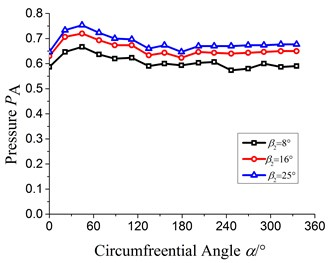a)$Q=$ 0.6${Q}_{d}$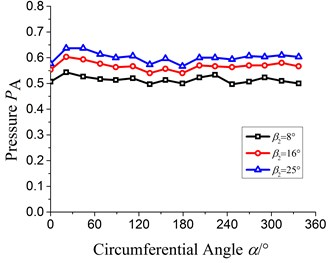b)$Q=$ 1.0${Q}_{d}$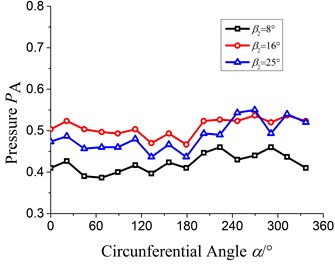c)$Q=$ 1.4${Q}_{d}$

Fig. 8The non-uniformity of static pressure at the impeller outlet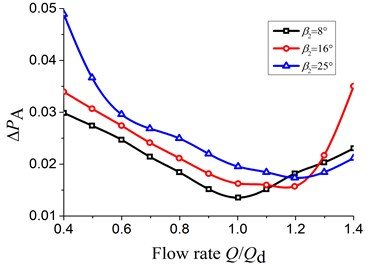### 3.3. The effect on the head pulsation

To study the effect of ${\beta }_{2}$ on the head pulsation, the test results of the head pulsation are shown in the Fig. 9. The horizontal coordinate $\theta$ represents the impeller rotation angle. When $\theta =$ 0°, 360° and 720°, the low pulse signal means the blade outlet just sweeps across the tongue.

It can be seen from the Fig. 9 that all head pulsation curves are similar under different flow rates and blade outlet angles. Also, all head pulsation curves change periodically with $\theta$. In each rotation circle, there are a maximum head and a minimum head.

Fig. 9Head pulsation in time domain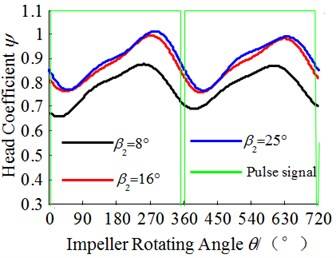a)$Q=$ 0.6${Q}_{d}$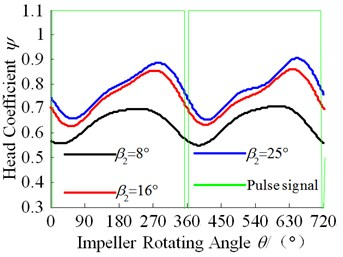b)$Q=$ 1.0${Q}_{d}$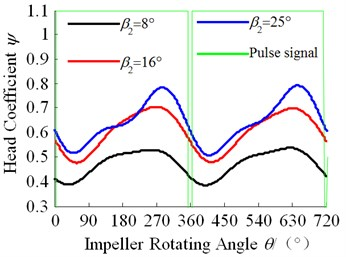c)$Q=$ 1.4${Q}_{d}$

What can be seen from the Fig. 9(a) is that the time domain of the maximum head and the minimum head is affected by the changing of ${\beta }_{2}$ and the impeller rotating angle corresponding to the minimum head at each ${\beta }_{2}$ respectively is 28°, 43° and 55°, and the rotating angle corresponding to the maximum respectively is 255°, 280° and 290°.

What is shown in the Fig. 9(b) is that the impeller rotating angle corresponding to the minimum head at each ${\beta }_{2}$ respectively is 30°, 50°, 62° and the rotating angle corresponding to the maximum head respectively is 247°, 278° and 289°.

As shown in Fig. 9(c), the impeller rotating angle corresponding to the minimum head at each ${\beta }_{2}$ respectively is 39°, 62°, 49.5° and the rotating angle corresponding to the maximum head respectively is 230°, 270°, 288°.

From the above analysis, the minimum head pulsation occurs between $\theta =$ 28°-62°, and the minimum head in time domain indicates gradually lag with the increasing of ${\beta }_{2}$. However, it doesn’t agree well with the former conclusion at large flow rate (1.4${Q}_{d}$), which needs to be explored carefully in the future. The maximum head pulsation occurs between $\theta =$ 230°-290° and it gradually advances with the increasing of ${\beta }_{2}$.

The explanation for above conclusion may be the below analysis. When ${\beta }_{2}$ increases, the fluid velocity at the impeller outlet increases and the angle between the fluid velocity and the casing tongue angle is positive incidence, and then the kinetic energy translates into the pressure after it collides with the fluid in the volute ahead. And then the high pressure domain moves to the upstream along the volute profile and this condition brings about that the head in time domain shows hysteresis.

More than above, the head of this pump increases with the increasing of ${\beta }_{2}$, which keeps in line with Fig. 6(a). Meanwhile the amplitude of the head pulsation shows the increasing trend, especially in large flow rate.

### 3.4. The effect on the pressure fluctuation

In order to analyze the pressure fluctuation quantitatively, the pressure fluctuation coefficient is defined as the following Eq. (2):

2
${P}_{A}^{*}=2\sqrt{\frac{T}{\mathrm{\Delta }\mathrm{t}}\sum _{j=0}^{T/\mathrm{\Delta }\mathrm{t}-1}{\left(p\left({t}_{0}+j\mathrm{\Delta }t\right)-\overline{p}\right)}^{2}}/\rho {u}_{2}^{2}.$

The Fig. 10 shows the pressure fluctuation of P2, P6, P10, and P14 under different flow rates.

The pressure fluctuation coefficient at P2 and P6 exits larger value under the small flow rate and is about 1.5 to 2 times under large flow rate. The pressure fluctuation coefficients get larger values under small and large flow rate at the P10 and P14. And compared to the large flow rate, the pressure fluctuation coefficient is small under the small flow rate; The maximum increasing amplitude of the pressure fluctuation coefficient in the four measuring points under the large flow rate respectively is 28.35 %, 22.4 %, 35.33 % and 40.0 % and the changing amplitude under the small flow rate respectively is 20.58 %, 23.11 %, 40.14 % and 13.6 %. Therefore, the maximum changing amplitude of the pressure fluctuation coefficient occurred at the P10 corresponding to the sixth section of the volute.

Fig. 10The pressure fluctuation at impeller outlet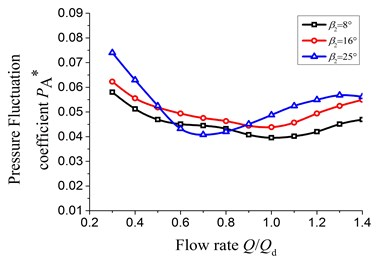a) P2 measuring point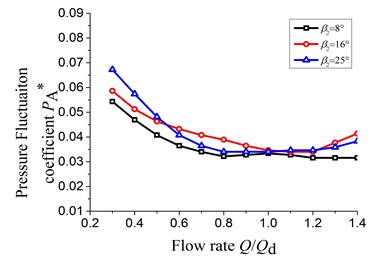b) P6 measuring point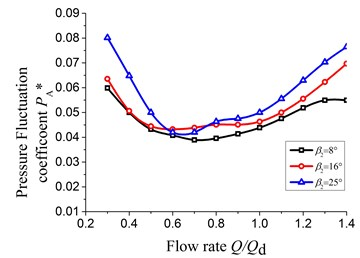c) P10 measuring point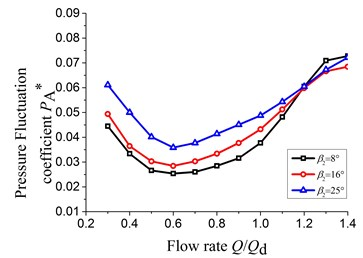d) P14 measuring point

Meanwhile the pressure fluctuation coefficients in each measuring point show the trend of increasing first and then decreasing with the increasing of the flow rate. The reason for this phenomenon is that absolute velocity angle of the fluid at the impeller outlet under small flow rate is not only less, but also positive incidence relative to the casing tongue angle. And then the velocity of fluid becomes bigger after flowing into the volute from the casing tongue and there will generate impulsion because of the difference in speed and direction between the high speed fluid flowing into the volute and the fluid in the volute. Therefore, the pressure fluctuation is more intense at P2 and P6 measuring points under small flow rate. Under the large flow rate, the flow velocity from the impeller outlet keeps accelerating along the flow direction and this velocity achieves maximum around at P10 and P14 measuring points. Therefore, the pressure fluctuation coefficient arise larger value at P10 and P14 measuring points in the volute.

### 3.5. The effect on the radial force

The radial force can be obtained by integration for the pressure of measuring points [14, 15] at impeller outlet along volute spiral channel and this can be considered as the reaction of fluid induced radial force in impeller. With this method, the measured radial force is the average value within a period of time, and defined as ${K}_{Rstat}$.

The effect of ${\beta }_{2}$ on the distribution of average radial force within a rotation period is presented in Fig. 11.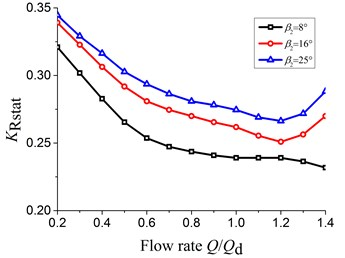With the increasing of flow rate from 0.2${Q}_{d}$ to 1.2${Q}_{d}$, the radial force decreases gradually. When the flow rate is bigger than 1.2${Q}_{d}$, the radial force under ${\beta }_{2}$ of 16° and 25° starts to increase. So, under ${\beta }_{2}$ of 16° and 25°, the minimum of radial force is all at 1.2${Q}_{d}$, which is about the best efficiency point. Consequently, when ${\beta }_{2}$ is equal to or bigger than 16°, the ${\beta }_{2}$ has no effect on position of minimum radial force.

When ${\beta }_{2}=$ 8°, the minimum radial force is 0.23. As the ${\beta }_{2}=$ 16° and ${\beta }_{2}=$ 25°, the minimum radial force is 0.25 and 0.26 respectively. Hence, with ${\beta }_{2}$ increasing, the minimum radial force also increases and the maximum increase amplitude is larger than 8 percentages.

What can be seen easily form the picture above is that as ${\beta }_{2}$ is equal to 8°, the radial force always decreases with the increasing of the flow rate. The reason may be that the load distribution at the blade outlet and the circumferential uniformity are small enough, which leads to radial force smaller. Meanwhile the flow separation and secondary flow can be suppressed since the ${\beta }_{2}$ is smaller, which causes the time-average radial force still decreases when the flow rate increases. Nevertheless when ${\beta }_{2}$ is equal to or bigger than 16°, the redial force represents first decrease and then increase.

On the other hand, the radial force increases at each flow rate condition with the increasing of ${\beta }_{2}$. The reason of the effect of ${\beta }_{2}$ on the radial force is that the circumferential uniformity of the static pressure at the impeller outlet becomes bigger, and meanwhile the increasing amplitude of the static pressure at the impeller shows the trend of deceasing with the increasing of the blade outlet angle. Because the radial force is obtained by integration ${\beta }_{2}$ agrees with the changing of the uniformity of the static pressure at the impeller outlet.

## 4. Conclusions

The effect of the ${\beta }_{2}$ on the energy and unsteady characteristics of the single channel pump have been studied via the synchronous testing method. Some conclusions can be concluded.

1) With the increasing of the ${\beta }_{2}$ from 8° to 25°, the head represents the gradually increasing and the maximum increase amplitude gets touch with 22.6 %. The efficiency has the maximum value and the maximum increase is 6.48 percentage points. The mixing loss occurring at the impeller outlet can be decreased by reducing the ${\beta }_{2}$.

2) With the increasing of ${\beta }_{2}$, the minimum head in time domain gradually lag and the maximum head gradually advances. And meanwhile the amplitude of the head pulsation shows the increasing trend, especially in large flow rate.

3) The pressure fluctuation coefficients in each measuring point show the trend of increasing first and then decreasing with the increasing of the flow rate and the maximum changing amplitude of the pressure fluctuation coefficient occurred at the P10 corresponding the sixth section of the volute.

4) The radial force increases as the ${\beta }_{2}$ increases. The changing of the radial force is related to the static pressure at impeller outlet, which has a significant effect on the radial force under each blade outlet angle.

#### Cited by

Study on the Unsteady Characteristics and Radial Force of a Single-Channel Centrifugal Pump

19 October 2016
Accepted
01 June 2017
Published
15 August 2017
SUBJECTS
Flow induced structural vibrations
Keywords
single channel pump